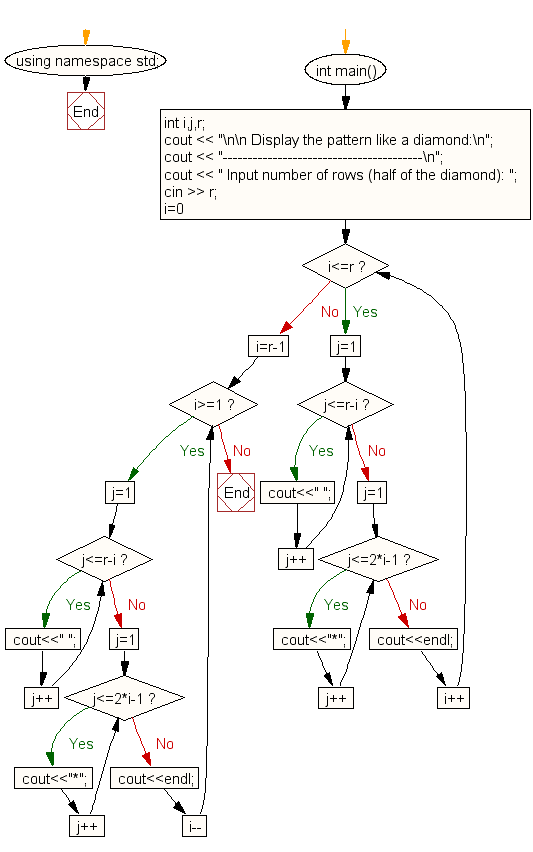﻿ C++ Exercises: Display the pattern like a diamond - w3resource

# C++ Exercises: Display the pattern like a diamond

## C++ For Loop: Exercise-44 with Solution

Write a C++ program to display a pattern like a diamond.

Sample Solution:

C++ Code :

``````#include <iostream>
using namespace std;

int main()
{
int i,j,r;
cout << "\n\n Display the pattern like a diamond:\n";
cout << "----------------------------------------\n";
cout << " Input number of rows (half of the diamond): ";
cin >> r;
for(i=0;i<=r;i++)
{
for(j=1;j<=r-i;j++)
cout<<" ";
for(j=1;j<=2*i-1;j++)
cout<<"*";
cout<<endl;
}
for(i=r-1;i>=1;i--)
{
for(j=1;j<=r-i;j++)
cout<<" ";
for(j=1;j<=2*i-1;j++)
cout<<"*";
cout<<endl;;
}
}
``````

Sample Output:

``` Display the pattern like a diamond:
----------------------------------------
Input number of rows (half of the diamond): 5

*
***
*****
*******
*********
*******
*****
***
*
```

Flowchart:C++ Code Editor: